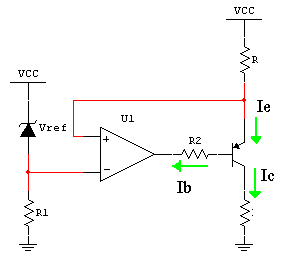# Electronic Device And Electronic Circuit

Data of electronic device , PCB Design and electronic circuitWeb basicelectronic.blogspot.com

## Friday, August 18, 2006

### Constant current source circuit

We can use a voltage reference turn into a Constant current source
circuit and use pnp-transistors for current-boostingBy op amp action ,the voltage across R is away Vref , give
Ie = Vref / R

Ie = Ib + Ic = (Ic/B) + Ic

Ic = [B/(B+1)] *Ie

Io = Ic = [B/(B+1)] * Vref / R about Vref / R
VL = Io*RL

Where maximum voltage of Vl(max) <>

We can adjust output current by adjust current setting resistance R